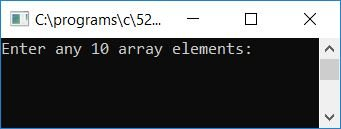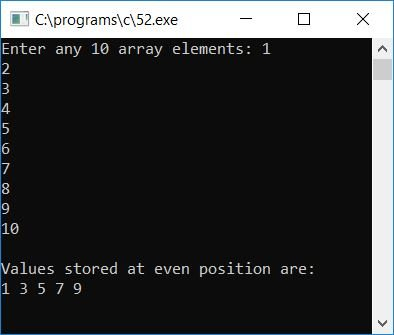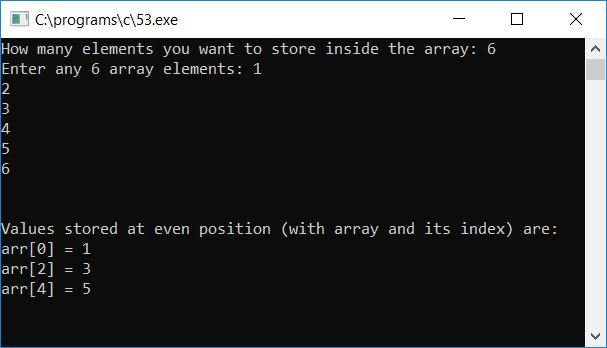# C Program to Print Array Elements Present in Even Position

In this article, we will learn how to create a program in C that will ask the user to enter array elements and print all the array elements present at even index numbers or even positions. Here is the program.

```#include<stdio.h>
#include<conio.h>
int main()
{
int arr, i;
printf("Enter any 10 array elements: ");
for(i=0; i<10; i++)
scanf("%d", &arr[i]);
printf("\nValues stored at even position are:\n");
for(i=0; i<10; i++)
{
if(i%2==0)
printf("%d ", arr[i]);
}
getch();
return 0;
}```

As the program was written in the Code::Blocks IDE, here is the first snapshot of the sample run:As we all know, the indexing in an array starts at 0, so here is the second snapshot of the sample run. The user has to provide any 10 numbers as 10 array elements for the given array and press the ENTER key to see the following output:Here is a list of some of the main steps used in the above program:

• Receive any 10 numbers as array elements.
• Create a for loop stars from the 0th number (array element) to the 9th number (array element).
• Inside the for loop, check whether the index number is an even number or not.
• If it is an even number, then print the value present at that even index number.
• In this way, we will see all the array elements present at an even index number on the output screen after running the program.

### Allow the user to define the array size

Here is the modified version of the above program. In this program, we have allowed the user to enter the size of the array at run-time:

```#include<stdio.h>
#include<conio.h>
int main()
{
int arr, i, limit;
printf("How many elements you want to store inside the array: ");
scanf("%d", &limit);
printf("Enter any %d array elements: ", limit);
for(i=0; i<limit; i++)
scanf("%d", &arr[i]);
printf("\n\nValues stored at even position (with array and its index) are:\n");
for(i=0; i<limit; i++)
{
if(i%2==0)
printf("arr[%d] = %d\n", i, arr[i]);
}
getch();
return 0;
}```

Here is the final snapshot of the sample run:Here are some of the main steps used in the above program:

• Receive the size of the array, say 20.
• Now receive any 20 numbers as 20 array elements.
• And then follow all the steps as told in the previous program.
• That is, find each even index number one by one in order to print all of the values stored at even index numbers.

C Quiz

« Previous Program Next Program »